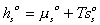# Bond Graph Modeling of Non-Electrolyte Gel

## 3. Overall Process: Mixing/Diffusion and Deformation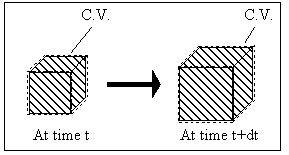System: (Polymer gel network + solvent inside) inside the control volume (c.v.)., i.e., the control volume is changing as the get network is expanding due to absorbing the surrounding solvent fluid.

Modeling Assumptions:

• Process is relatively slow, and the inertial effects of fluid flow and polymer network are negligible.
• Process is solvent diffusion-limited into the gel network, and the effects of surrounding fluid convection is negligible.
• Process is quasistatic; i.e., the properties inside the c.v. is uniform at any instant of time.
• Process is isothermal (constant temperature), isobaric (constant pressure), which is typical in laboratory setting.
• Process consists of two sub-processes: mixing/diffusion and elastic expansion of the polymer-solvent network (Flory).
• The polymer and solvent are incompressible.

## Mixing/Diffusion Process: Entropy generating process

Consider the polymer gel network inside the c.v. as shown above. The c.v. is surrounded by the solvent fluid at constant temperature, To, and constant pressure, Po. As the solvent fluid enters the c.v., the c.v. expands, and the state of the system inside the control volume at an instant of time can be themodynamically described by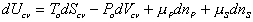(1)

where

• Ucv is the internal energy of the system inside the c.v.,
• Scv is the entropy of the system inside the c.v.,
• Vcv is the volume of the c.v.,
• To is the temperature the system inside the c.v. experiences,
• Po is the pressure the system inside the c.v. experiences,
•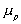is the chemical potential of polymer inside the c.v.,
•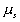is the chemical potential of solvent inside the c.v.,
• np is the number of mole of polymer inside the c.v.,
• ns is the number of mole of solvent inside the c.v.

Since the number of mole of polymer inside the c.v. is fixed, the third term on the right hand side (RHS) is zero, i.e.,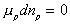. In addition, the second term on the RHS, PodVcv, represents the differential expansion work done by the c.v.. Assuming both the polymer and the solvent to be incompressible, then PodVcv = 0 since the expansion of c.v. alone is exactly cancelled by the flow work done by the solvent flowing into the c.v.. The Equation (1) then simplies to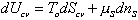(2)

With the assumption of quasi-staticity of the process, then the system inside the c.v. may be treated as the lumped parameter multiport capacitance with the relation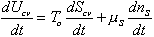(3)

The first term on RHS represents the effects of heat transfer, entropy transfer due to convection and the entropy generation due to irreversibility. The second term on RHS represents the chemical energy brought in by the incoming surrounding solvent. The bond graph representation of the system is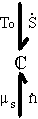The constitutive relations describing the equilibrium state of the polymer-solvent gel network system has been derived by Flory, and it will be base of the multiport capacitance model.

### Flory's model: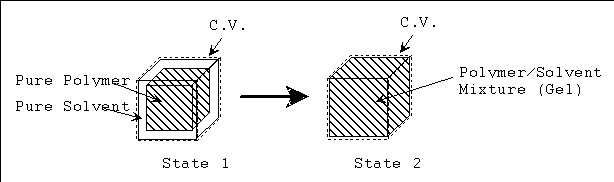• State 1: The pure polymer network and pure solvent inside the control volume (c.v.) are completely separated.

• State 2: The polymer network and the solvent inside the control volume mix and form homogeneous polymer solution, while maintaining constant volume of c.v.

The process from state 1 to state 2 is therefore entropy generating run-down mixing process, and the changes in thermodynamic properties of control volume due to such mixing process are described by Flory.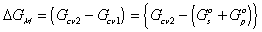where
•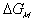is the change in Gibbs free energy of c.v. due to mixing,
• Gcv1 is the Gibbs free energy of c.v. at state 1,
• Gcv2 is the Gibbs free energy of c.v. at state 2,
• Gso is the standard Gibbs free energy of solvent,
• Gpo is the standard Gibbs free energy of polymer.

Similarly,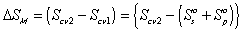where S denotes entropy.

The Gibbs free energy change of c.v. by mixing of polymer and solvent is considered to be due to two effects; combinatorial effect,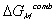, and polymer-solvent contact effect,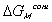.accounts for the many different conformations the amorphous polymer may adopt, andaccounts for the effects of non-randomness induced by intermolecular interactions.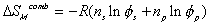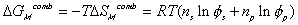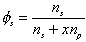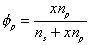where
• R is the universal gas constant,
• ns is the number of mole of solvent,
• np is the number of mole of polymer,
•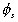is the volume fraction of solvent,
•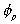is the volume fraction of polymer,
• x is the number of chain segments of a polymer molecule such that the volume of a polymer molecule is equal to x time the volume of a solute molecule, i.e.,

Vp = x Vs.

The intermolecular interaction also involves enthalpy change.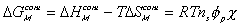where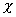is the Flory-Huggins polymer-solvent interaction paramter.is a temperature dependent dimensionless quantity which characterizes polymer-solvent interactions, and can be expressed in the form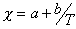where a and b are temperature independent quantities. Then,can be written as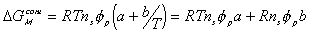Since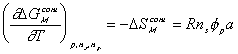then,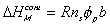Note that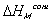represents the thermal energy generated due to the mixing process, and thus can be modelled as the heat transferred to the surrounding solvent environment while maintaining isothermal condition.

Combining both the combinatorial and polymer-solvent contact effects, we have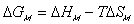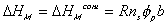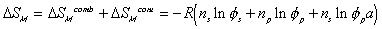The change in Gibbs energy can be viewed as the energy lost due to entropy generating process, and for quasistatic mixing/diffusion process, the rate of change ofcan be written as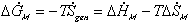Also,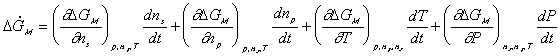Since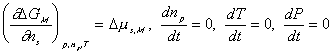it simplifies to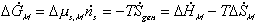This entropy generation process can be modelled as a 3-port resistance field driven by the chemical potentials of solvents across the gel's control volume boundary.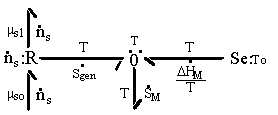Since the entropy and Gibbs free energy of gel system inside the control volume at are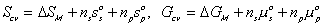and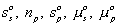are constant during the process, the rate of change of Scv, and Gcv with respect to time are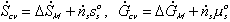Combining the above results, we then have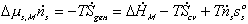The bond graph structure of the polymer-solvent mixing/diffusion model is therefore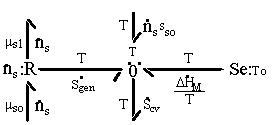Note that the resistive R field in the bond graph is presented such that the kinetics of the solvent flow may depend on both the chemical potentials of solvent outside and inside the gel rather than just the net difference in chemical potential across the gel boundary. The phenomenological description of diffusion/mixing process is also needed here to relate the rate of solvent flow into the control volume to the chemical potentials of solvent. For initial investigation, a simple description of non-electrolyte solvent diffusion is employed;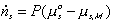where P represents the permeability coefficient.

## Rubber Elasticity

### Flory's model

According to Flory's work, deformation of polymer gel network may be modelled as consisting of two step processes; first, expansion due to free swelling and second, stretching at constant volume, without changing the enthalpy of the gel system. Based on statistical theory of rubber elasticity where the end-to-end distances of the polymer chains can be described by the Gaussian distribution, the Gibb's free energy change,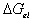, and the entropy change,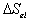, due to elastic deformation are described by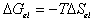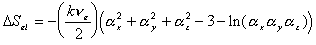where
• k is the Boltzmann's constant,
•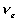is the effective number of chains in the gel,
•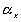represents the elongation factor in x-axis;= length/free length in x-axis
•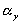represents the elongation factor in y-axis
•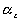represents the elongation factor in z-axis.

Assume that the network formed at volume Vo is subsequently swollen isotropically by a diluent to a volume V such that the volume fraction of polymer is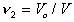. In the subsequent deformation due to stretching, the volume is assumed constant. Letting,, andrepresent the changes in dimensions resulting from the combination of swelling and elongation, we have therefore that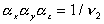is constant during the elongation. Considering only a simple elongation in the x-direction, let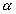represent the length in this direction relative to the swollen, unstretched length Lo,s = (V/Vo)1/3 Lo, since the swelling is isotropic, i.e.,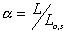Then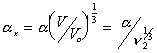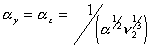Substituting the above results, then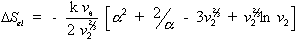For the deformation process during swelling and stretching, the heat of deformation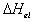is considered negligible, and therefore the Gibbs free energy is then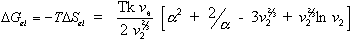At contant temperature as assumed previously,is a function of the number of mole of solvent in the c.v. and the elongation, i.e.,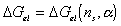. Taking the above result by Flory, then the rate of change of Gibb's free energy with respect to time can be represented as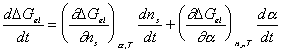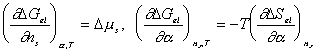and,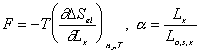After combining the above result and little algebraic manipulations, we have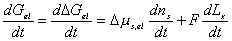and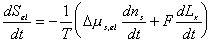Since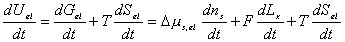the bondgraph structure for multiport capacitance due to rubber elasticity is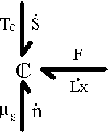The validity of the Flory's assumption of modelling the deformation process as two step process should be checked. Specificially, researchers have observed that elongation of elastomeric polymer gel increases the swelling degree of the gel. In other words, stretching decreases the osmotic pressure of solvent inside the gel whereas compressing has reverse effect. Similarly, it also implies that the chemical potential of the solvent inside the gel should decrease when stretched and increase when compressed. However, it is found that the Flory's model does not predict such behavior according to the our preliminary results, but in fact predicts reverse effect. The validity of our results as well as the Flory's model of deformation effect need to be investigated further.

### Simple Mechanical Model of the Rubber Elasticity Effect

The above theory on rubber elasticity of swelling gel by Flory is based on the complex statistical theory which remarkably relates how the molecular structure of elastomer responds to an applied strain to the macroscopic deformation behavior. However, this theory still offers only qualitative approximation to the actual behavior of elastomer, mainly due to the assumption that end-to-end distances of the chains can be described by the Gaussian distribution. Given such deficiency of statistical model, the purpose of this section is to describe the gel's elasticitic behavior with simpler mechanical analog; the springs.

Let's consider a unit cube of the isotropic gel where the opposite faces of the cube are linked by a mechanical spring in all three axes. These mechanical springs represents the rubber elasticity of the gel elastomer, and therefore their rest lengths are the linear dimensions of gel when it is fully contracted and free of external stress. In addition, the elastomer itself occupies a constant finite volume. Defining the control volume as the space occupied by the solvent only, the mechanical springs inside the control volume then may be treated as volume-less pure mechanical spring elements, and let's assume such control volume is still an isotropic cube for simplicity. Then, static force balance at each face of the control volume relates the pressures of solvent to the tensile force as

Px Ax = Po Ax + Fx
Py Ay = Po Ay + Fy
Pz Az = Po Az + Fz
Ax = Ly Lz
Ay = Lx Lz
Az = Lx Ly

where
• Ax is the surface area of the face pointing in x-axis,
• Ay is the surface area of the face pointing in y-axis,
• Az is the surface area of the face pointing in z-axis,
• Px is the pressure exerted on Ax by solvent inside c.v.,
• Py is the pressure exerted on Ay by solvent inside c.v.,
• Pz is the pressure exerted on Az by solvent inside c.v.,
• Po is the external solvent bath pressure,
• Fx is the tensile force in x-axis,
• Fy is the tensile force in y-axis,
• Fz is the tensile force in z-axis,
• Lx is the elongation is x-axis,
• Ly is the elongation is y-axis,
• Lz is the elongation is z-axis.

Assuming the uniform pressure P inside the control volume,

Px = Py = Pz = P

and modeling the mechanical spring as simple Hookean spring, the spring forces are then

Fx = K Lx
Fy = K Ly
Fz = K Lz

where K is the spring stiffness which may be linear or nonlinear.

For the simple case of uniaxial tension in x-axis, Ly = Lz due to isotropy of gel, and thus

V = Lx Ly Lz = Lx Ly2

where V is the volume of the solvent inside the control volume.

Combining above results, we get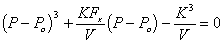where the solvent pressure P can be solved as a function of Fx and V.

Modeling the effect of rubber elasticity as purely mechanical, the change in chemical potential of solvent due to the rubber elasticity is then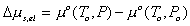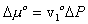where v1o is molar volume of pure solvent. Combining above two results, we get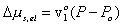For the case of free swelling, the effect of rubber elasticity is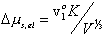and this result is consistent with Flory and Treloar.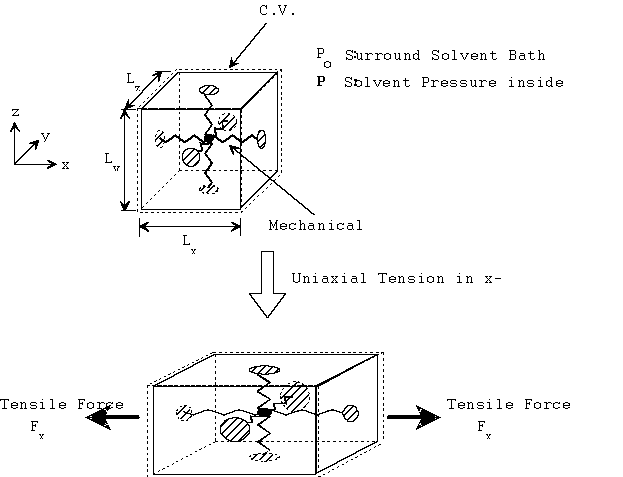Figure: Mechanical model of isotropic gel elastomer under uniaxial tension.

According to above results based on simple mechanical spring, the effect of elongation/compression on the swelling degree of gel is in agreement with the observed behavior. The change in chemical potential of solvent inside the gel is directly proportional to the osmotic pressure, and both the solvent's chemical potential and osmotic pressure is decreased when elongated and increased when compressed. Before proceeding, however, one should note the limitations of this simple mechanical model, namely the lack of coupling between the mechanics and thermodynamics of the mechanical spring. With real elastomer, there is thermal-mechanical energy conversion, as Flory's model describes in terms of entropy change due to deformation. For instance, when the elastomer is stretched, it is observed to grow warmer. Such energy coupling behavior is neglected in this simple pure mechanical spring model, and the importance of this coupling effect needs to be investigated further in order to validate this simple model.

## Overall Process: Mixing/Diffusion and Deformation

Combining the previous results on mixing/diffusion process and the deformation process, we then have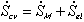and, therefore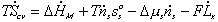where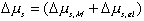One should note that the mechanical force contribution in above equation is based on Flory's rubber elasticity model, and it does not show up explicitly in junction structure since it is embedded in the nature of multiport capacitance. Applying the integral causality to chemical and mechanical ports to the multiport capacitance field, the bond graph representation of the overall system is then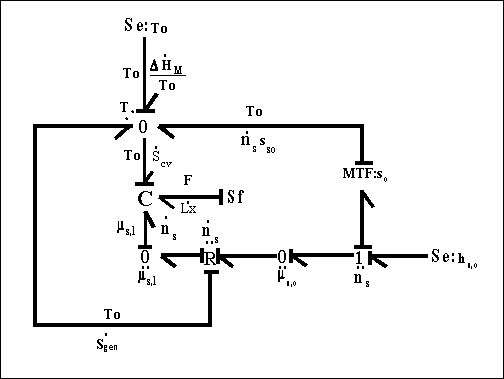Note that for the surrounding solvent